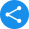# NCERT Class 1 Maths Book PDFView Notes

Mathematics, as a subject helps students learn problem-solving skills. Students can practise questions from NCERT Books for Class 1 Maths and strengthen their fundamental concept of Mathematics. Major boards like CBSE and other state boards prescribe Class 1 Maths NCERT Book for students as these help them build the basis for future study. Students can read from the NCERT 1st Class Maths Book syllabus and practice the questions to excel in their exam. These exercise questions are exam-oriented and help students significantly.

 Class 1st Board CBSE Book NCERT Subject Maths Medium English / Hindi Study Materials Free Study Materials Available PDF Solutions Available in PDF Part 1 NCERT Books Class 1 Maths PDF Download

We have curated solutions for the books that you follow which you can access from the below links.

### NCERT Solutions for Class 1

1. Why Learning Maths is Essential for Class 1 Student?

Ans. Solving math questions ensure the improvement of analytical and reasoning capabilities of a student. They will be able to learn and perform simple calculations such as addition, subtraction, multiplication, etc. needed in day to day life. Learning these concepts will help students solve problems quickly and efficiently.

2. How to Learn Solve Mathematical Questions?

Ans. Students can learn the basic concept behind a chapter and practise them to solve the questions accurately. In this endeavour, NCERT Books for Class 1 Maths can be beneficial for students as they provide the right concept and steps needed to learn it.

3. How Can I Learn a Chapter if I Miss a Class in School?

Ans. It might happen with any students that they end up missing a critical class, and this can cause trouble before the exam. In such cases, they can look for live classes from Vedantu and quickly learn the concepts and practise questions.

4. What are Some Important Chapters in Mathematics for Class 1?

Ans. Few critical chapters from exam point of view for students of Class 1 can be – Addition, subtraction, numbers, time, data handling, measurement, etc. Students need to pay attention in class and refer to their NCERT books for understanding the concepts effectively.

Share this with your friendsShare